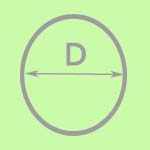Turf Calculator

How do I calculate the amount of turf I need in my garden?
How do I know how much turf to order?
What is the area of my garden?

Here is the answer to all your questions: This is a turf calculator which can help to ensure you order the correct amount of turf for your garden.

To use the calculator just enter the width and length of your garden into box A and B, you can select metres, yards or feet.
Then select "calculate" for an instant sq meterage of your garden. We always work in square metres - remember 1 roll = 1 sqm

### Rectangle Calculator

 Measurements in: Meters Feet Yards A =   X   B =    Total Area = m�### Circle Calculator

 Measurements in: Feet Yards Metres Circle Diameter    Total Area = m�